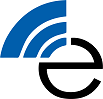## Mobile and Wireless Propagation

#### Discover how wireless propagation works and what are concepts exploited in the solution of real-world wireless applicative problems### Course Content

##### Fundamentals of electromagnetic fields

Maxwell equations. Constitutive relations of the medium. SW/HW-emulator exercise: "Determination of the dielectric constant of samples under test". Initial and boundary conditions. SW/HW-emulator exercise: "Testing the law of power decrease (in anechoic chamber)". Classification of electromagnetic problems. Energy theorems (time domain). Uniqueness theorem (time domain).

##### Elementary solutions and interactions

Plane waves: plane waves in dissipative media, plane waves in anisotropic media. Plane waves at the discontinuity: spatial discontinuities, temporal discontinuities, lossy media, guided waves. SW exercise: "FDTD analysis of electromagnetic phenomena related to the incidence of an em wave on a metallic plane".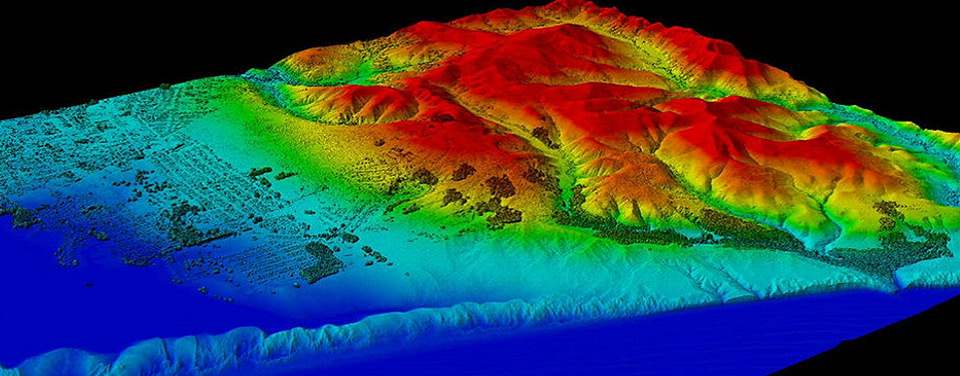##### Plane waves in phasor domain

Vector wave equation. D'Alembert vector equation. Helmholtz equation. Wave functions. Propagation of plane waves. Polarization states. Secondary constants of the medium. Spectrum of plane waves. Guided propagation: (a) normal incidence on flat interface (dielectric-dielectric, dielectric-conductor, (b) oblique incidence on dielectric half-space. SW/HW-emulator exercise: "Analysis of the interference pattern caused by reflections from a metallic plane".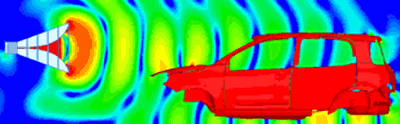##### Transmission lines and circuit theory

Elementary theory of the transmission lines. Characteristic equations of the transmission lines (time domain). Propagation of a signal along a uniform transmission line (time domain). Telegraph equation. Solution of the transmission lines equations (phasor domain). SW/HW-emulator exercise: "Measurement of the wavelength with a slotted line". Impedance, admittance and reflection coefficients. SW/HW-emulator exercise: "Impedance measurement with a slotted line". Definition of standing wave. Diagram of standing wave. Voltage standing wave ratio. SW/HW-emulator exercise: "Measurement of the reflection coefficient and of the VSWR with a slotted line". Using the formalism of the transmission lines for the study of the reflection of uniform plane waves. Smith chart. SW exercise: "Using the Smith chart (SW SMITHPRO program)".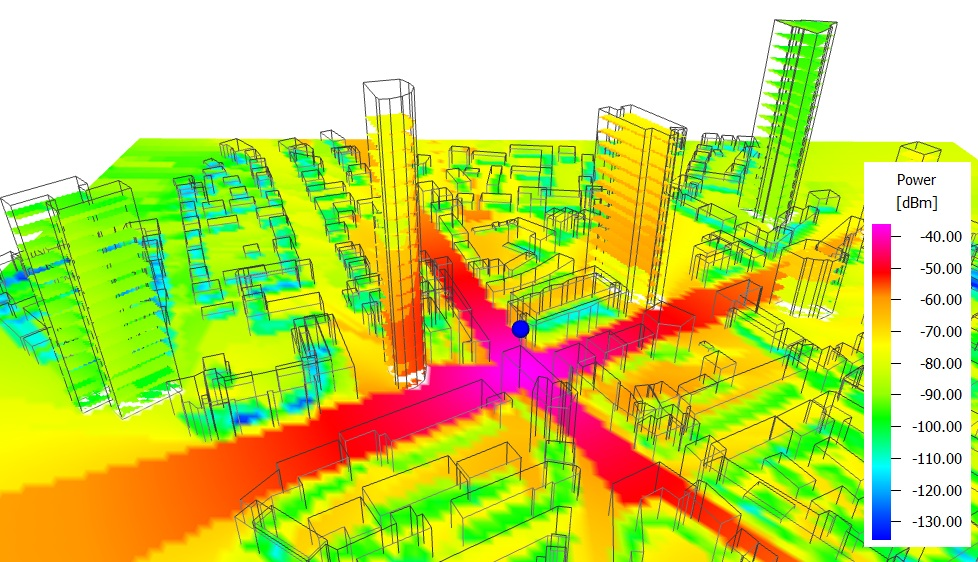• G. Franceschetti, "Electromagnetics. Theory, Techniques, and Engineering Paradigms", Ed: Kluwer Academic/Plenum Publishers, 1997.
• G. Conciauro, "Introduzione alle onde elettromagnetiche", Ed: Mc-Graw-Hill, 1993.
• J. Stratton, "Electromagnetic Theory", Ed. McGraw-Hill, 1941.
• F. Morichetti and A. Melloni, “Mezzi di trasmissione per l'informazione”, Ed: Independently Published, 2018.
• F. Morichetti and A. Melloni, “Mezzi di trasmissione per l'informazione: Esercizi svolti”, Ed: Independently Published, 2018.

• Teacher:
• Syllabus:
• Material:
• Course:
• Institution
• Language:
ItalianFunctionality of an automotive radar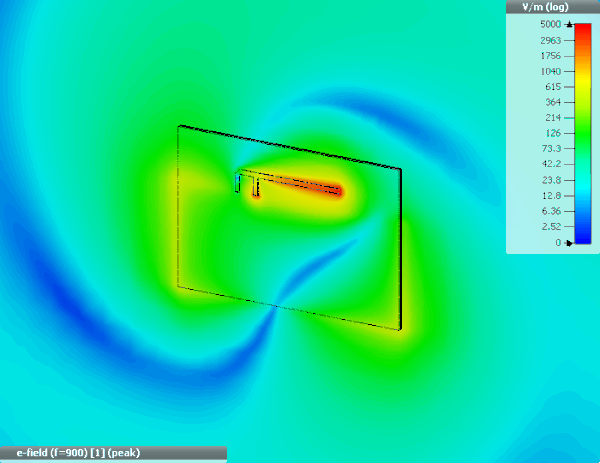5G smartphone antenna simulation